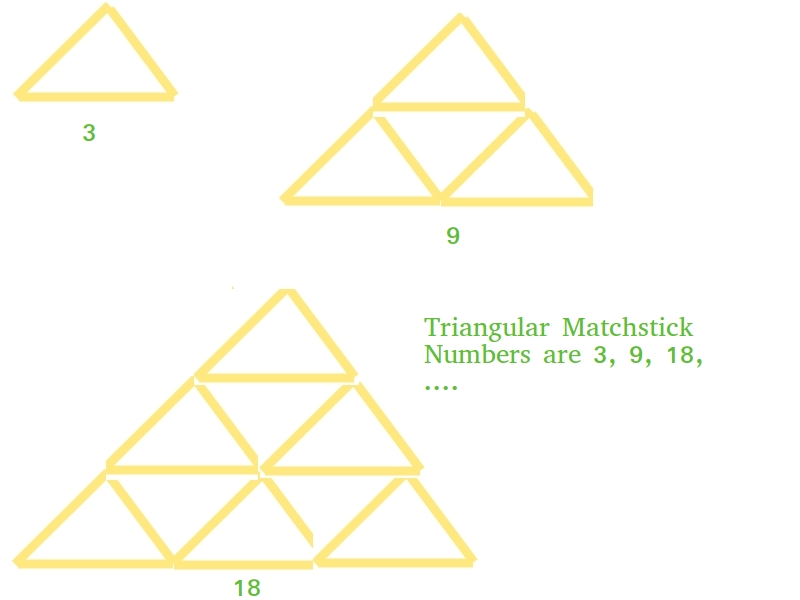# Triangular Matchstick Number

Given a number X which represents the floor of a matchstick pyramid, write a program to print the total number of matchstick required to form pyramid of matchsticks of x floors.

Examples:

```Input : X = 1
Output : 3
Input : X = 2
Output : 9
```

## Recommended: Please try your approach on {IDE} first, before moving on to the solution.

This is mainly an extension of triangular numbers. For a number X, the matchstick required will be three times of X-th triangular numbers, i.e., (3*X*(X+1))/2## C++

 `// C++ program to find X-th triangular ` `// matchstick number ` ` `  `#include ` `using` `namespace` `std; ` ` `  `int` `numberOfSticks(``int` `x) ` `{ ` `    ``return` `(3 * x * (x + 1)) / 2; ` `} ` ` `  `int` `main()  ` `{ ` `    ``cout<

## Java

 `// Java program to find X-th triangular ` `// matchstick number ` `public` `class` `TriangularPyramidNumber { ` `    ``public` `static` `int` `numberOfSticks(``int` `x) ` `    ``{ ` `        ``return` `(``3` `* x * (x + ``1``)) / ``2``; ` `    ``} ` `    ``public` `static` `void` `main(String[] args) ` `    ``{ ` `        ``System.out.println(numberOfSticks(``7``)); ` `    ``} ` `} `

## Python3

 `# Python program to find X-th triangular ` `# matchstick number ` ` `  `def` `numberOfSticks(x): ` `    ``return` `(``3` `*` `x ``*` `(x ``+` `1``)) ``/` `2` `     `  `# main() ` `print``(``int``(numberOfSticks(``7``))) `

## C#

 `// C# program to find X-th triangular ` `// matchstick number ` `using` `System; ` ` `  `class` `GFG ` `{ ` `    ``// Function to ind missing number ` `    ``static` `int` `numberOfSticks(``int` `x) ` `    ``{ ` `        ``return` `(3 * x * (x + 1)) / 2; ` `    ``} ` ` `  `    ``public` `static` `void` `Main() ` `    ``{ ` `        ``Console.Write(numberOfSticks(7)); ` `    ``} ` `} ` ` `  `// This code is contributed by _omg `

## PHP

 ` `

Output:

```84
```

Attention reader! Don’t stop learning now. Get hold of all the important DSA concepts with the DSA Self Paced Course at a student-friendly price and become industry ready.

My Personal Notes arrow_drop_upCheck out this Author's contributed articles.

If you like GeeksforGeeks and would like to contribute, you can also write an article using contribute.geeksforgeeks.org or mail your article to contribute@geeksforgeeks.org. See your article appearing on the GeeksforGeeks main page and help other Geeks.

Please Improve this article if you find anything incorrect by clicking on the "Improve Article" button below.

Improved By : jit_t

Article Tags :
Practice Tags :

Be the First to upvote.

Please write to us at contribute@geeksforgeeks.org to report any issue with the above content.